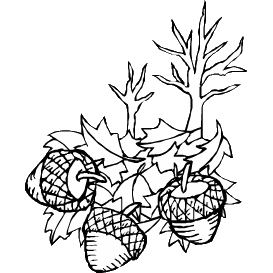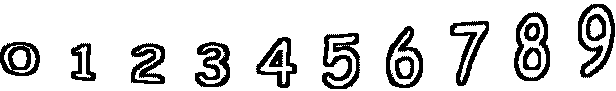1 + 2 =        10 + 8 =6 + 4 =        1 + 8 =        2 + 9 =        6 + 0 = 2 + 8 =        1 + 7 =        8 + 2 =        10 + 0 =